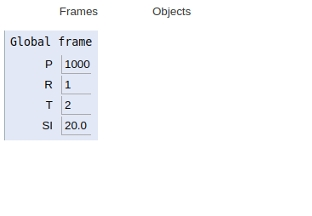# Simple interest in Python Program

PythonServer Side ProgrammingProgramming

#### Beyond Basic Programming - Intermediate Python

Most Popular

36 Lectures 3 hours

#### Practical Machine Learning using Python

Best Seller

91 Lectures 23.5 hours

#### Practical Data Science using Python

22 Lectures 6 hours

In this article, we will learn about the calculation of simple interest in Python 3.x. Or earlier.

## Simple interest

is calculated by multiplying the daily interest rate by the principal amount by the number of days that elapse between the payments.

Mathematically,

Simple Interest = (P x T x R)/100

Where,

P is the principal amount

T is the time and

R is the rate

For Example,

If P = 1000,R = 1,T = 2

Then SI=20.0

Now let’s see how we can implement a simple interest calculator in Python.

## Example

Live Demo

P = 1000
R = 1
T = 2
# simple interest
SI = (P * R * T) / 100
print("simple interest is", SI)

## Output

simple interest is 20.0


Here the simple interest is obtained three arithmetic products and one arithmetic division.

Now let’s see the scope of the variables declared. As shown in the figure below all variables are declared in the global scope.## Conclusion

In this article, we learnt about simple interest and it’s implementation using Python script.